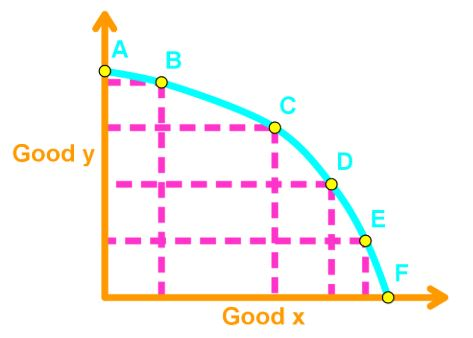# Resource efficiency

### Resource efficiency

#### Lessons

In this section, we will learn how to pick the best choice in the PPF.

PPF and Marginal Cost

Recall that a point is production efficient when it is on the PPF. However, which point on the PPF is the best choice?We need to see which point gives the greatest benefit.

Allocative efficiency is gained when the goods and services are produced at the lowest cost and gives the greatest benefit. In other words, a choice at allocative efficiency is the best option.

Marginal Cost: the opportunity cost of producing one additional unit. This can be calculated by finding the slope of two near points along the PPF.

Recall the slope formula to be:

m = $\large \frac{y_{2} \, - \, y_{1}}{x_{2} \, - \, x_{1}}$

By calculating all slopes between two points along PPF, we can use those slopes to create a marginal cost curve.

Marginal Benefit: is the benefit received from consuming one more unit of it. Benefits are subjective and depends on people’s preferences.

Note: Marginal Benefits cannot be derived from the PPF and is usually given in the question.

Finding the Allocative Efficiency

When MB > MC, then the benefit of an additional unit is higher than the cost of an additional unit. So, you would want the additional unit because the marginal net benefit is positive.

When MB < MC, then the benefit of an additional unit is less than the cost of an additional unit. So, you would not want the additional unit because the marginal net benefit is negative.

Therefore, the allocative efficiency exists when MB = MC. This is because you don’t gain anything from the additional unit, so there is no point.

• Introduction
Resource Efficiency Overview:
a)
PPF and Marginal Cost
• Allocative Efficiency
• Defining Marginal Cost
• Creating the Marginal Cost Curve

b)
Marginal Benefit
• Benefit received from one additional unit
• Willingness to Pay
• Cannot be derived from PPF

c)
Finding the Allocative Efficiency
• MB = MC
• An example of Finding Allocative Efficiency